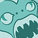# App Development

Announcements
Now Live: Qlik Sense SaaS Simplified Authoring – Analytics Creation for Everyone: READ DETAILS
cancel
Showing results for
Did you mean:Contributor II

## ABC Analysis

Hi All,

I am trying to build a dashboard on ABC analysis, where we classify the materials as Category A (80%), Category B(15%) and Category C (5%).

In the filter pane, I am trying to show a Range % which should show how much % (ex 10%, 20%, 30% etc upto 100%), total unique materials, unique suppliers is contributing to the respective Range %,. If i select upto 50%, it should show all the material details until 50% i.e upto 10%+20%+30%+40%+50%.

I tried the following in the data load editor but it is not giving the correct result.

if("Total %"<=10,'upto 10%', if("Total %">10 and "Total %"<=20, 'upto 20%', if("Total %">20 and "Total %"<=30, 'upto 30%',
if("Total %">30 and "Total %"<=40, 'upto 40%', if("Total %">40 and "Total %"<=50, 'upto 50%', if("Total %">50 and "Total %"<=60, 'upto 60%',
if("Total %">60 and "Total %"<=70, 'upto 70%', if("Total %">70 and "Total %"<=80, 'upto 80%', if("Total %">80 and "Total %"<=90, 'upto 90%',
if("Total %">90 and "Total %"<=100, 'upto 100%')))))))))) as range

i tried this also but no correct results.

if(rangesum(peek("Percentage %"),rowno())<=10.00,'upto 10%',
if(rangesum(peek("Percentage %"),rowno())>10.00 and rangesum(peek("Percentage %"),rowno())<=20.99,'upto 20%',
if(rangesum(peek("Percentage %"),rowno())>20.00 and rangesum(peek("Percentage %"),rowno())<=30.99,'upto 30%',
if(rangesum(peek("Percentage %"),rowno())>30.00 and rangesum(peek("Percentage %"),rowno())<=40.99,'upto 40%',
if(rangesum(peek("Percentage %"),rowno())>40.00 and rangesum(peek("Percentage %"),rowno())<=50.99,'upto 50%',
if(rangesum(peek("Percentage %"),rowno())>50.00 and rangesum(peek("Percentage %"),rowno())<=60.99,'upto 60%',
if(rangesum(peek("Percentage %"),rowno())>60.00 and rangesum(peek("Percentage %"),rowno())<=70.99,'upto 70%',
if(rangesum(peek("Percentage %"),rowno())>70.00 and rangesum(peek("Percentage %"),rowno())<=80.99,'upto 80%',
if(rangesum(peek("Percentage %"),rowno())>80.00 and rangesum(peek("Percentage %"),rowno())<=90.99,'upto 90%',
if(rangesum(peek("Percentage %"),rowno())>90.00 and rangesum(peek("Percentage %"),rowno())<=100.99,'upto 100%')))))))))) as Range%

I have attached the data file for your reference.

Regards

Vani

Labels (1)
• ### General Question

1 Solution

Accepted SolutionsChampion III

As classification can change based user selections this make more sense to be calculated in chart and not in script, as then the classifications would be static

refer below

https://community.qlik.com/t5/Qlik-Design-Blog/Recipe-for-an-ABC-Analysis/ba-p/1472867

Vineeth Pujari
If a post helps to resolve your issue, please accept it as a Solution.Champion III

As classification can change based user selections this make more sense to be calculated in chart and not in script, as then the classifications would be static

refer below

https://community.qlik.com/t5/Qlik-Design-Blog/Recipe-for-an-ABC-Analysis/ba-p/1472867

Vineeth Pujari
If a post helps to resolve your issue, please accept it as a Solution.Tags